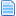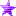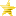Started by -Extasis-, November 18, 2013, 05:43:10 pm

-Extasis-Bar Fluid Increase and Degreaded Progressively
#1  November 18, 2013, 05:43:10 pm
••• Tu tiempo paso, tu ya no brilla'♪♫
Code:
`[State 1500,            VarSet]type                    = VarSet;adjust you,depends on the size you use explodtrigger1                = 1v                       = 30value                   = 3000[State 1500,            VarAdd]type                    = VarAddtrigger1                = Var(40)>fvar(31)trigger2                = Var(40)<fvar(31)fv                      = 31value                   = ((Var(40)-Fvar(31))/2)[State 1500,            VarSet]type                    = VarSettrigger1                = 1v                       = 40value                   = Playerid(56),power[State 1500,            VarSet]type                    = VarSettrigger1                = 1fv                      = 32value                   = (fvar(31)/var(30))`

power bars, health bars, systems bars, but fluent, I mean it grows slowly
you can AdjuStar the growth rate in the division of FVAR 31
Use variables to divide, because mugen does not divide into one formulates

good luck
yo confio en que vuelvas a ser mi princesa ♥ ♪♫